# Important Questions for SSC (Marathi Semi-English) 10th Standard [इयत्ता १० वी] - Maharashtra State Board - Geometry

Subjects
Topics
Subjects
Popular subjects
Topics
Geometry
< prev  1 to 20 of 518  next >

In the following figure, in ΔPQR, seg RS is the bisector of ∠PRQ. If PS = 6, SQ = 8, PR = 15, find QR.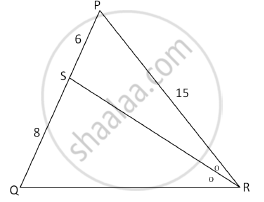Appears in 3 question papers
Chapter: [0.01] Similarity
Concept: Similarity of Triangles

In the following figure RP: PK= 3:2, then find the value of A(ΔTRP):A(ΔTPK).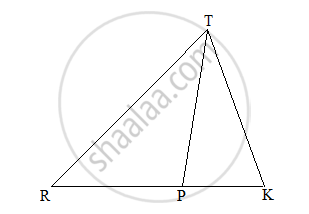Appears in 3 question papers
Chapter: [0.01] Similarity
Concept: Properties of Ratios of Areas of Two Triangles

Prove that ‘the opposite angles of a cyclic quadrilateral are supplementary’.

Appears in 3 question papers
Chapter: [0.03] Circle

If two circles with radii 8 cm and 3 cm, respectively, touch internally, then find the distance between their centers.

Appears in 3 question papers
Chapter: [0.03] Circle
Concept: Touching Circles

A person standing on the bank of river observes that the angle of elevation of the top of a tree standing on the opposite bank is 60°. When he moves 40 m away from the bank, he finds the angle of elevation to be 30°. Find the height of the tree and width of the river. (sqrt 3=1.73)

Appears in 3 question papers
Chapter: [0.06] Trigonometry
Concept: Heights and Distances

Using Euler’s formula, find V if E = 30, F = 12.

Appears in 3 question papers
Chapter: [0.07] Mensuration
Concept: Euler's Formula

Find the diagonal of a square whose side is 10 cm.

Appears in 3 question papers
Chapter: [0.07] Mensuration
Concept: Circumference of a Circle

Find the diagonal of a square whose side is 10 cm.

Appears in 3 question papers
Chapter: [0.07] Mensuration
Concept: Circumference of a Circle

In Fig. 3, AP and BP are tangents to a circle with centre O, such that AP = 5 cm and ∠APB = 60°. Find the length of chord AB.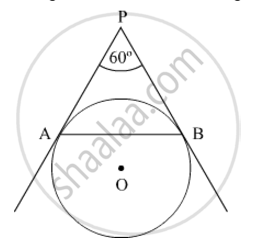Appears in 3 question papers
Chapter: [0.07] Mensuration
Concept: Areas of Sector and Segment of a Circle

A farmer connects a pipe of internal diameter 20 cm from a canal into a cylindrical tank which is 10 m in diameter and 2 m deep. If the water flows through the pipe at the rate of 4 km per hour, in how much time will the tank be filled completely?

Appears in 3 question papers
Chapter: [0.07] Mensuration
Concept: Surface Area of a Combination of Solids

In the following figure seg AB ⊥ seg BC, seg DC ⊥ seg BC. If AB = 2 and DC = 3, find (A(triangleABC))/(A(triangleDCB))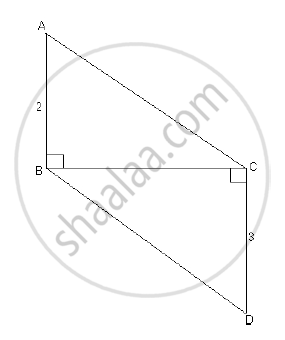Appears in 2 question papers
Chapter: [0.01] Similarity
Concept: Properties of Ratios of Areas of Two Triangles

Prove that the angle bisector of a triangle divides the side opposite to the angle in the ratio of the remaining sides.

Appears in 2 question papers
Chapter: [0.01] Similarity
Concept: Similarity of Triangles

In the following figure, seg BE ⊥ seg AB and seg BA ⊥ seg AD. If BE = 6 and $\text{AD} = 9 \text{ find} \frac{A\left( \Delta ABE \right)}{A\left( \Delta BAD \right)} \cdot$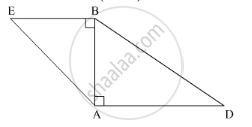Appears in 2 question papers
Chapter: [0.01] Similarity
Concept: Similarity of Triangles

In the given figure, AD is the bisector of the exterior ∠A of ∆ABC. Seg AD intersects the side BC produced in D. Prove that :

$\frac{BD}{CD} = \frac{AB}{AC}$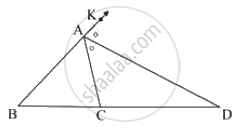Appears in 2 question papers
Chapter: [0.01] Similarity
Concept: Properties of Ratios of Areas of Two Triangles

In ∆PQR, point S is the midpoint of side QR. If PQ = 11, PR = 17, PS = 13, find QR.

Appears in 2 question papers
Chapter: [0.02] Pythagoras Theorem
Concept: Right-angled Triangles and Pythagoras Property

Some question and their alternative answer are given.

In a right-angled triangle, if sum of the squares of the sides making right angle is 169 then what is the length of the hypotenuse?

Appears in 2 question papers
Chapter: [0.02] Pythagoras Theorem
Concept: Apollonius Theorem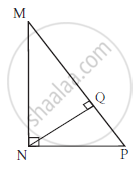In ΔMNP, ∠MNP = 90˚, seg NQ ⊥ seg MP, MQ = 9, QP = 4, find NQ.

Appears in 2 question papers
Chapter: [0.02] Pythagoras Theorem
Concept: Right-angled Triangles and Pythagoras Property

Prove that “The lengths of the two tangent segments to a circle drawn from an external point are equal.”

Appears in 2 question papers
Chapter: [0.03] Circle
Concept: Number of Tangents from a Point on a Circle

Prove that “The lengths of the two tangent segments to a circle drawn from an external point are equal.”

Appears in 2 question papers
Chapter: [0.03] Circle
Concept: Number of Tangents from a Point on a Circle

If two circles with radii 5 cm and 3 cm respectively touch internally, find the distance between their centres.

Appears in 2 question papers
Chapter: [0.03] Circle
Concept: Touching Circles
< prev  1 to 20 of 518  next >
Important Questions for SSC (Marathi Semi-English) 10th Standard [इयत्ता १० वी] Maharashtra State Board Geometry. You can further filter Important Questions by subjects and topics. Chapter wise important Questions for 10th Standard [इयत्ता १० वी] Maharashtra State Board. it gets easy to find all 10th Standard [इयत्ता १० वी] important questions with answers in a single place for students. Saving time and can then focus on their studies and practice. Important questions for 10th Standard [इयत्ता १० वी] chapter wise with solutions

10th Standard [इयत्ता १० वी] Geometry can be very challenging and complicated. But with the right ideas and support, you will be able to make it work. That’s why we have the 10th standard [इयत्ता १० वी] Geometry important questions with answers pdf ready for you. All you need is to acquire the PDF with all the content and then start preparing for the exam. It really helps and it can bring in front an amazing experience every time if you're tackling it at the highest possible level.

We make sure that you have access to the important questions for 10th Standard [इयत्ता १० वी] Geometry S.S.C Maharashtra State Board. This way you can be fully prepared for any specific question without a problem. There are a plethora of different questions that you need to prepare. And that's why we are covering the most important ones. They are the questions that will end up being more and more interesting and the results themselves can be staggering every time thanks to that. The quality itself will be quite amazing every time, and you can check the important questions for 10th Standard [इयत्ता १० वी] Geometry Maharashtra State Board whenever you see fit.

The important questions for 10th Standard [इयत्ता १० वी] Geometry 2021 we provide on this page are very accurate and to the point. You will have all the information already prepared and that will make it a lot simpler to ace the exam. Plus, you get to browse through these important questions for 10th Standard [इयत्ता १० वी] Geometry 2021 S.S.C and really see what works, what you need to adapt or adjust and what still needs some adjustment in the long run. It's all a matter of figuring out these things and once you do that it will be a very good experience.

All you have to do is to browse the important questions for upcoming Maharashtra State Board 10th Standard [इयत्ता १० वी] 2021 on our website. Once you do, you will know what needs to be handled, what approach works for you and what you need to do better. That will certainly be an incredible approach and the results themselves can be nothing short of staggering every time. We encourage you to browse all these amazing questions and solutions multiple times and master them. Once you do that the best way you can, you will have a much higher chance of passing the exam, and that's what really matters the most!